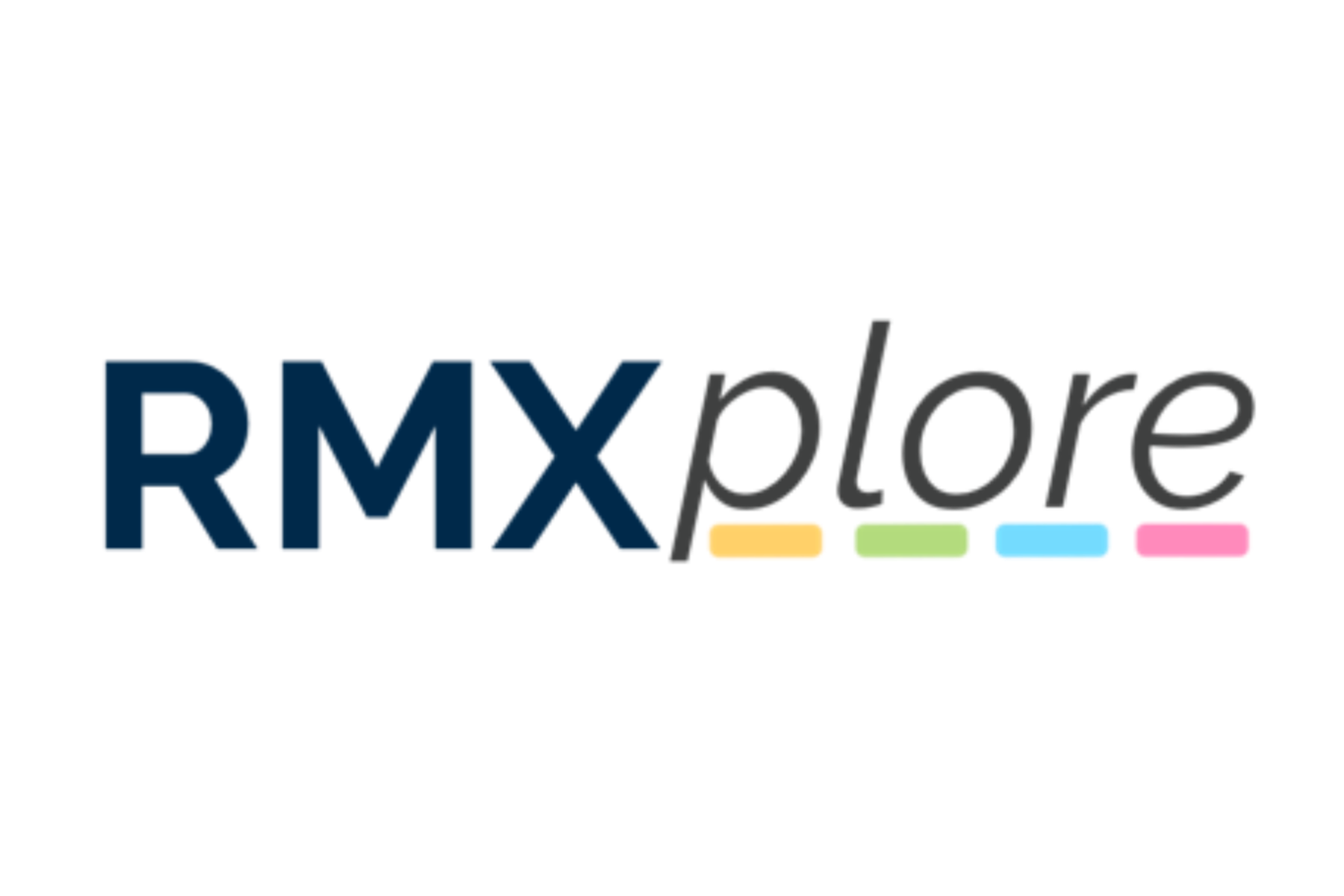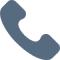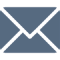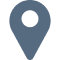## Prealgebra

### Prealgebra

Begins September 16th, 2023
Course Pricing:
• Online : \$1695
• Cupertino Center : \$1695
Course Logistics:
Duration : 120 minutes
Number of Classes : 30 Classes
Saturdays (12:00 - 2:00 p.m.)
<< Back To Courses

### Course Topics:

Course Topics are organized as six modules with five granular topics covered under each module. Click through below to see all modules/topics for this course.

### 01 – Review of Arithmetical Operations• Whole numbers – Operations
• Exponents
• Fractions, Decimals, and Percentages
• Ratio and Proportions

### 02 – Applications of Arithmetics• Word problems involving exponents and radicals
• Problem solving using percentage (profit/loss, mixture, etc.)
• Ratio and Proportions (age, population, etc.)
• Rates (Interest, Speed, Work)

### 03 - Introduction to Number Theory• Prime numbers
• Divisibility rules
• Prime factorization
• Multiples and Least Common Multiple (LCM)
• Divisors and Greatest Common Divisor (GCD)

### 04 - Introduction to Algebra• Expressions
• Linear Equations - 1
• Linear Equations - 2
• Inequalities – 1
• Word problems involving linear equations and inequalities

### 05 - Introduction to Geometry• Angle chasing
• Perimeter and area of shaded regions
• Triangles and PythagoreanTheorem
• Lateral/total surface area and volume of 3D objects

### 06 - Introduction to Counting• Basic Statistics
• Venn Diagrams
• Counting with Addition and Subtraction
• The Multiplication Principle
• Introduction to Probability